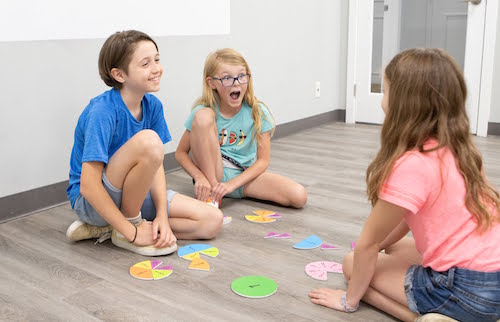To succeed in higher-level mathematics, students must develop math fluency – the ability to automatically retrieve facts and perform essential calculations. Once they feel confident in their own mastery of math fundamentals, students begin to experiment, take risks, and make new conceptual connections.

RRLS math classes are designed to engage students as they develop math fluency in a supportive environment. Abstract concepts are made concrete with engaging activities, manipulatives, and exploratory play. By the end of each program, students will feel proud of the independence they have gained.

## Early Math Group Classes#### Grades 1 to 3 – Mathemagics

Mathemagics is an active, engaging program designed to unleash students’ developing numeracy skills! Based on Number Sense strand of Ontario’s Grade 2 mathematics curriculum, this class offers students the chance to develop their math confidence in a supportive environment. Students entering Grade 2 will have the chance to work ahead, while students entering Grades 3 and 4 will revisit areas of challenge and build independence.

In this ten-hour program, students will explore:

Skip-counting by 2s, 5s, 10s, and 25s
Mental math strategies for addition and subtraction
Place value
2-digit addition and subtraction with and without regrouping
Reading and representing money amounts of to 100 cents

#### Grades 4 to 6 – Power Solvers

Power Solvers is a dynamic program designed to boost student confidence with word problems! Many students find word problems challenging and this course will cover both how to understand the word problem and do the math to solve it.

This course is most appropriate for students in grades 4 to 6. Solving the word problems in this course will involve addition, subtraction, multiplication, and division with whole numbers, decimals, and fractions.  Students will be allowed to use calculators to check their work.

In this six-hour program, students will learn how to:

Break down word problems with the CUBES strategy
Identify the keywords and necessary operations
Model word problems with drawings, diagrams, and tables
Answer word problems with complete sentences

## Get Started!